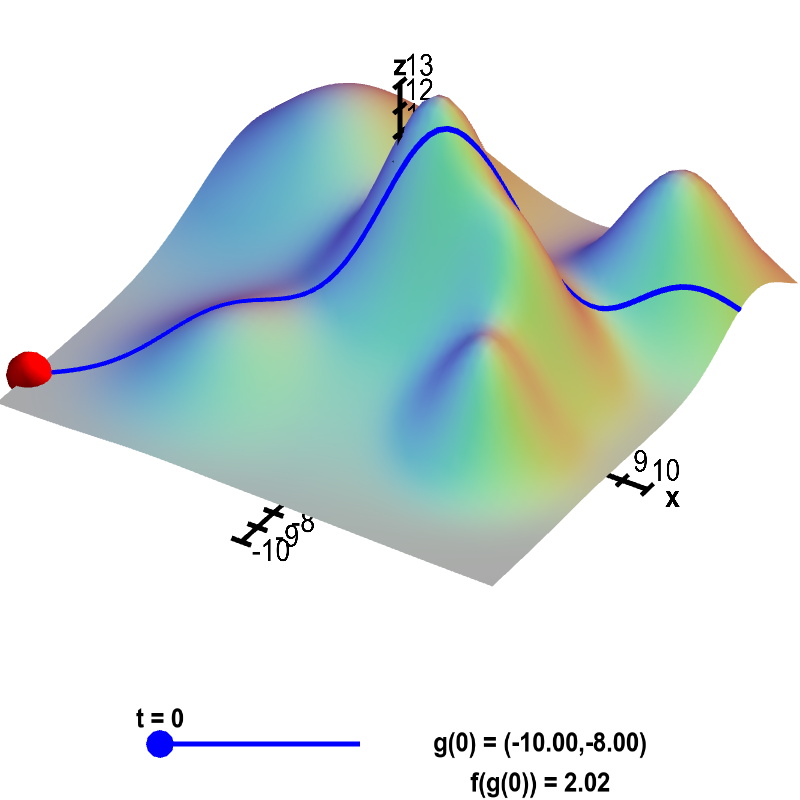# Math Insight

### Applet: Crossing a mountain rangeAn illustration of a path through a mountain range. The height $f(x,y)$ of a mountain range at position $(x,y)$ is shown by the surface plot. A path through the mountains is shown by the blue curve. The $xy$-coordinates along the path at time $t$ are given by the function $\vc{g}(t)=(g_1(t),g_2(t))$. The blue curve is drawn to be raised at the height the mountain range $f(\vc{g}(t))$ so that it appears to be describing the position of someone walking through the mountain range. For any value of $t$ (changeable via the slider), the $x$ and $y$-coordinates of the red point are $\vc{g}(t)$, and its height is $f(\vc{g}(t))$, as shown in the lower right. In other words, the coordinates of the red point are $(g_1(t),g_2(t),f(g_1(t),g_2(t))$, and the blue curve is the set of all those points.